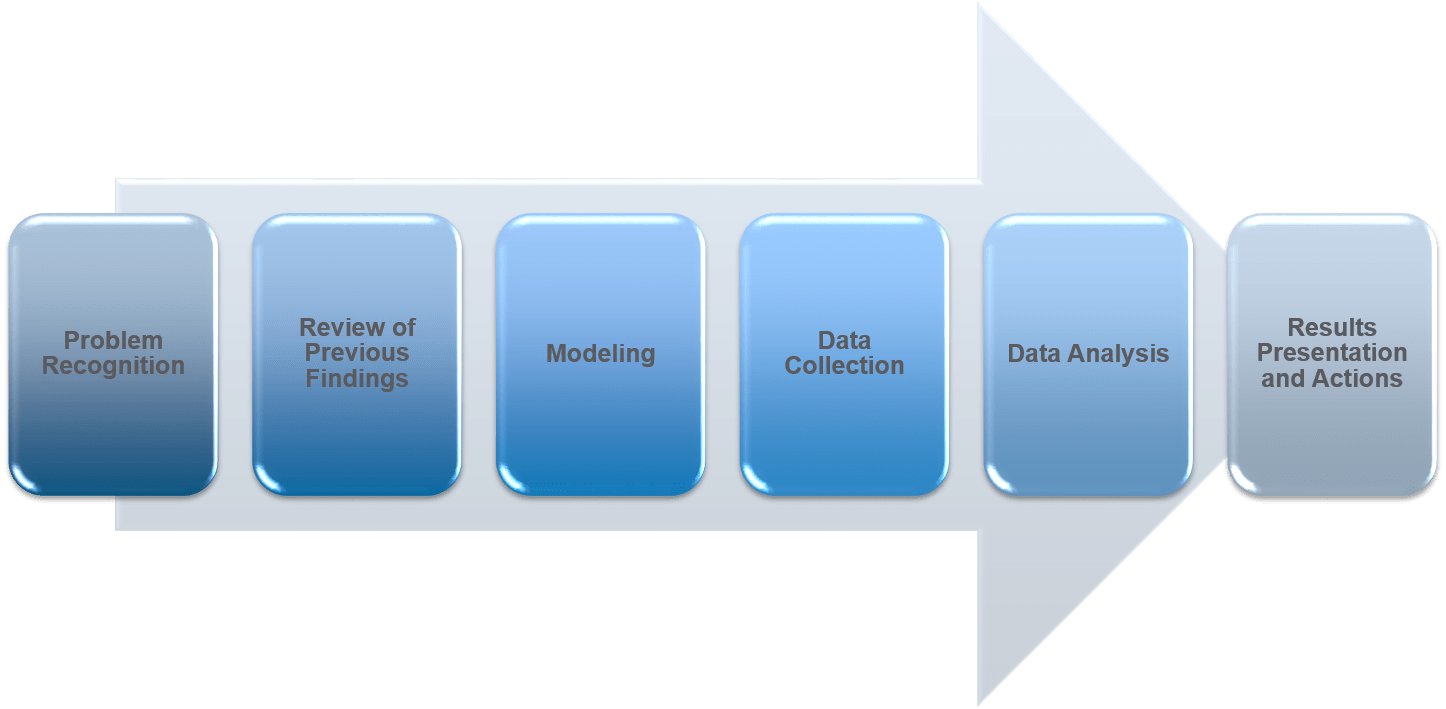# Comparing Machine Learning Models in Determining Credit Worthiness for Bank Loans

The R language has a number of machine learning libraries to help determine for both supervised and unsupervised machine learning. This includes such ML techniques such as linear and logistic regression, decision trees, random forest, generalized boosted regression modeling among others. I strongly recommend learning how these models work and how they can be used to predictive analytics.

Part of the Machine Learning process includes the following:

1. Sample: Create a sample set of data either through random sampling or top tier sampling.  Create a test, training and validation set of data.
2. Explore: Use exploratory methods on the data.  This includes descriptive statistics, scatter plots, histograms, etc.
3. Modify:  Clean, prepare, impute or filter data.  Perform cluster analysis, association and segmentation.
4. Model:  Model the data using Logistic or Linear regression, Neural Networking, and Decision Trees.
5. Assess:  Access the model by comparing it to other model types and again real data. Determine how close your model is to reality.  Test the data using hypothesis testing.

When creating machine learning models for any application, it is wise to following a process flow such as the following:In the following example, we use machine learning to determine the credit worthiness of prospective borrowers for a bank loan.

The loan data consist of the following inputs

1. Loan amount
2. Interest rate
4. Employment length of borrower
5. Home ownership status
6. Annual Income
7. Age of borrower

The response variable or predictor to predict the default rate

1. Loan status (0 or 1).

After loading the data into R, we partition the data for training or testing sets.

``````loan <- read.csv("loan.csv", stringsAsFactors = TRUE)

str(loan)

## Split the data into 70% training and 30% test datasets

library(rsample)
set.seed(634)

loan_split <- initial_split(loan, prop = 0.7)

loan_training <- training(loan_split)
loan_test <- testing(loan_split)
``````

Create a over-sample training data based on ROSE library. This checks for over-sampling of the data.

``````str(loan_training)

table(loan_training\$loan_status)

library(ROSE)

loan_training_both <- ovun.sample(loan_status ~ ., data = loan_training, method = "both", p = 0.5)\$data

table(loan_training_both\$loan_status)``````

Build a logistic regression model and a classification tree to predict loan default.

``````loan_logistic <- glm(loan_status ~ . , data = loan_training_both, family = "binomial")

library(rpart)

loan_ctree <- rpart(loan_status ~ . , data = loan_training_both, method = "class")

library(rpart.plot)

rpart.plot(loan_ctree, cex=1)
``````

Build the ensemble models (random forest, gradient boosting) to predict loan default.

``````library(randomForest)

loan_rf <- randomForest(as.factor(loan_status) ~ ., data = loan_training_both, ntree = 200, importance=TRUE)

plot(loan_rf)

varImpPlot(loan_rf)

library(gbm)

``````

``````loan_gbm <- gbm(loan_status ~ ., data = loan_training_both, n.trees = 200, distribution = "bernoulli")
summary(loan_gbm)

``````

Use the ROC (receiver operating curve) and compute the AUC (area under the curve) to check the specificity and sensitivity of the models.

``````# Step 1. Predicting on test data

predicted_logistic <- loan_logistic %>%
predict(newdata = loan_test, type = "response")

predicted_ctree <- loan_ctree %>%
predict(newdata = loan_test, type = "prob")

predicted_rf <- loan_rf %>%
predict(newdata = loan_test, type = "prob")

predicted_gbm <- loan_gbm %>%
predict(newdata = loan_test, type = "response")

# Step 3. Create ROC and Compute AUC

library(cutpointr)

roc_logistic <- roc(loan_test, x= .fitted_logistic, class = loan_status, pos_class = 1 , neg_class = 0)
roc_ctree<- roc(loan_test, x= .fitted_ctree, class = loan_status, pos_class = 1 , neg_class = 0)
roc_rf<- roc(loan_test, x= .fitted_rf, class = loan_status, pos_class = 1 , neg_class = 0)
roc_gbm<- roc(loan_test, x= .fitted_gbm, class = loan_status, pos_class = 1 , neg_class = 0)

plot(roc_logistic) +
geom_line(data = roc_logistic, color = "red") +
geom_line(data = roc_ctree, color = "blue") +
geom_line(data = roc_rf, color = "green") +
geom_line(data = roc_gbm, color = "black")

auc(roc_logistic)
auc(roc_ctree)
auc(roc_rf)
auc(roc_gbm)``````

These help you compare and score which model works best for the type of data presented in the test set. When looking at the ROC chart, you can see that the gradient boost model has the best performance of all the model as it is closer to 1.00 than the other models. Classifiers that are closer to 1.00 for the top left where Sensitivity is 1.00 and Specificity is closer to 0.00 have the best performance.